# Let's Get Started

One way to learn how to multiply and factor trinomials is to use algebra tile models.

TEKS Standards and Student Expectations

A(8) Quadratic functions and equations. The student applies the mathematical process standards to solve, with and without technology, quadratic equations and evaluate the reasonableness of their solutions. The student formulates statistical relationships and evaluates their reasonableness based on real-world data. The student is expected to:

A(8)(A) solve quadratic equations having real solutions by factoring, taking square roots, completing the square, and applying the quadratic formula

A(8)(B) write, using technology, quadratic functions that provide a reasonable fit to data to estimate solutions and make predictions for real-world problems

Resource Objectives(s)

Use models to multiply factors.

Use models to factor quadratic equations.

Essential Questions

How can models be used to multiply factors?

How can models be used to factor a quadratic equation?

How can factors be used to find the solutions to a quadratic equation?

Vocabulary

# Multiplying Binomials Using Tiles

Example 1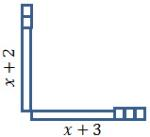The tiles in this example show a model of (x + 2)(x + 3). The length and the width represent the factors.

The long rectangular tiles represent the x-variable:

• The vertical rectangular tile represents the x-variable in the first factor.
• The horizontal rectangular tile represents the x-variable in the second factor.

The square tiles represent the constant:

• The two square tiles above the vertical rectangle are the constant 2.
• The three square tiles next to the horizontal rectangle are the constant 3.

When we put the x and the constant together, we get the two factors:

• The first factor is (x + 2).
• The second factor is (x + 3).

Example 2

Click "Start animation" and watch as the tiles below are moved to create an algebraic tile diagram of (x − 2)(x + 1).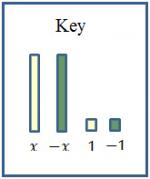Example 3

Click "Start animation" and watch as the tiles below are moved to create an algebraic tile diagram that will be used to find the product of (x − 1)(x − 2).To find the product of (x − 1)(x − 2):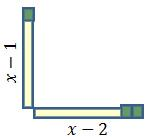B. Draw a vertical and horizontal line on the inside of the horizontal and vertical tiles.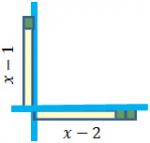C. Draw horizontal lines extending the ends of the original vertical tiles.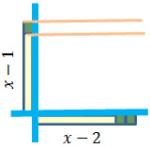D. Draw vertical lines extending the ends of the original horizontal tiles. The object is to create a rectangle with the length and width of the factors of the trinomial.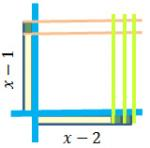Step 1: The large red square tile is $x2$.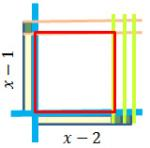Step 2:  The small unit square tile in the upper left corner is shaded to show a negative; therefore, the entire row is shaded in.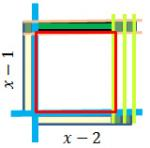Step 3: The small unit square tiles in the bottom right are shaded to show a negative number; therefore, the last two columns are shaded in. But the top two unit square tiles in the upper right hand corner are already shaded.

Remember that a negative multiplied by a negative is a positive. Since the shaded areas are negative numbers and the top two unit squares are already shaded, they would change to not shaded because the process is the same as a negative multiplied by a negative number, or no shading.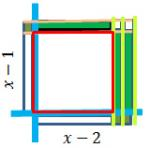Step 4: The last step is reading the diagram.

• The large square tile =$X2$
• The three long shaded rectangular tiles =$-3x$
• The two small square tiles = $2$

Therefore, the product of (x − 1)(x − 2) is: $x2-3x+2$.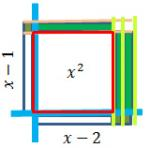Answer the following two questions to check your understanding on multiplying binomials using tiles.

# Factoring Trinomials

Algebra tiles can also be used to factor trinomials.

The following directions explain how to find the factors of .

A. Create a rectangular diagram with the tiles for the trinomial.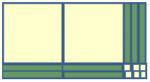B. Find the factors from the rectangular diagram.

• There are two large squares; therefore, one of the x's has a coefficient of 2.

• There are three green shaded rectangular columns of tiles on the right side, so the constant in the first factor is -3.

• There are two rows of green shaded rectangular tiles at the bottom, so the constant in the second factor is -2.

• The factors of  are .

# Using Tiles to Find Solutions to Quadratic Equations

Now let's use all the information we've covered to solve a quadratic equation based on the configuration of the algebraic tiles. To solve a quadratic equation, you must first set the equation equal to zero. The Zero Factor Principle tells us that at least one of the factors must be equal to zero. Since at least one of the factors must be zero, we will set them each equal to zero:

Example 1:

Solve .

The tile diagram would look like this: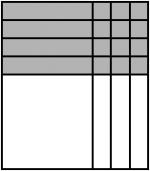The factors are .

Since we are solving the equation, set the product equal to zero.

To solve  , set each factor equal to 0.

The solution to the equation : is  and .

If x is replaced in the original equation with -3, the equation is true.

If x is replaced in the original equation with 4, the other value for x, the equation is true.

Now watch as tiles are used to create a diagram.

Follow the steps to solve the equation.